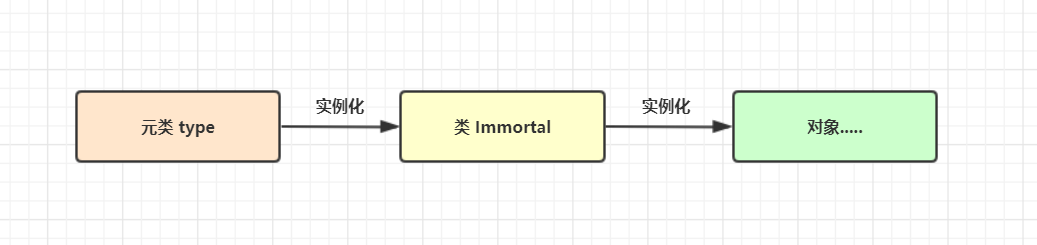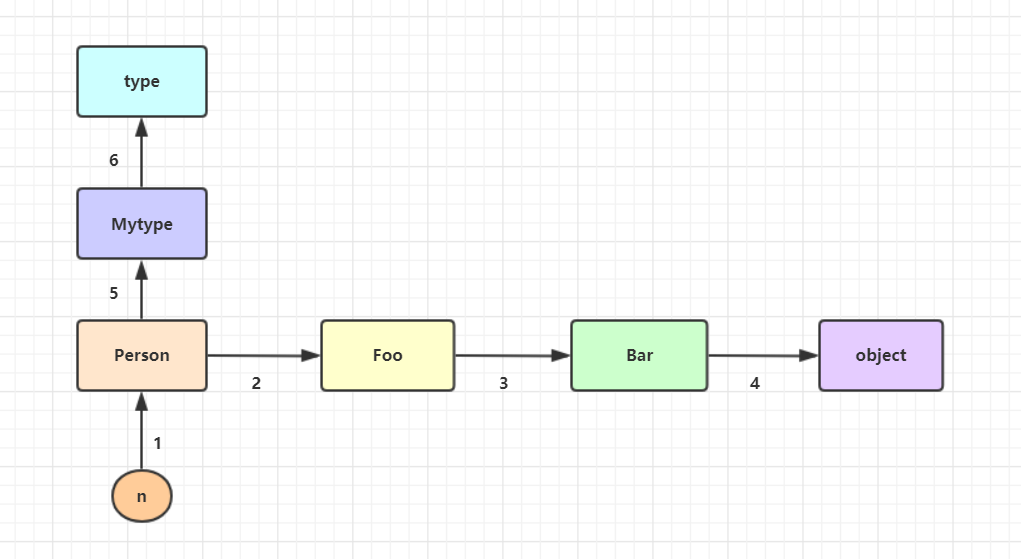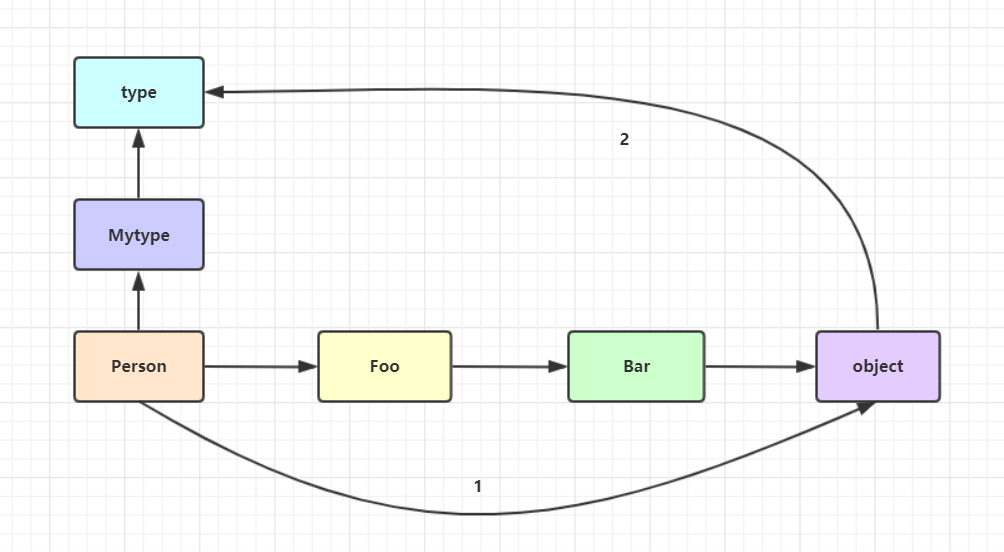# 71 元类(黑魔法)

22次阅读

## 引入

Python 中一切皆对象, 那么 本质上也是一个对象## 一. 什么是元类

### 1. 先定义一个类来进行分析

``````class Immortal(object):
def __init__(self,name,age):
self.name = name
self.age = age

p = Immortal(" 太白 ",4555)
print(type(p))  # <class '__main__.Immortal'>
``````

``````print(type(Immortal))  # <class 'type'>
print(type(type))      # <class 'type'>
``````## 二. 分析 class 关键字创建类的过程

### 1. 内置函数 exec 的用法

• 第一个参数是包含的要执行的一系列 Python 代码(字符串格式)
• 第二个参数是全局名称空间 (字典形式), 默认为 globals()
• 第三个参数是局部名称空间 (字典形式), 默认为 local()

``````msg = '''
name = "shawn"
age = 22
dic = {"sex":"man"}
def test():
print("I am shawn")
'''
globals_dic = {}  # 全局名称空间
locals_dict = {}  # 局部名称空间
exec(msg,globals_dic,locals_dict)

print(locals_dict)
# {'name': 'shawn', 'age': 22, 'dic': {'sex': 'man'}, 'test': <function test at 0x000002596D9BC4C8>}
print(locals_dict["name"])  # shawn
locals_dict["test"]()       # I am shawn
``````

### 2. 调用 type + ()来实现创建类

• 类名 : Immortal = type()
• 父类们(基类们) : class_bases = (object ,)
• 类的名称空间 : class_namespace (类的名称空间是执行类体代码而得到的)

``````💠设置类名
class_name = "Immortal"

💠设置基类们
class_bases = (object,)

💠设置类体(类的名称空间)
class_body = '''
name = "shawn"
age = 22
def print_name(self):
print(f"I am {self.name}")
'''
class_namespace = {}

💠运行 "exec" 函数
exec(class_body,{},class_namespace)

💠创建类
Immortal = type(class_name,class_bases,class_namespace)

💠实例化
p = Immortal()
print(p.name,p.age)  # shawn 22
p.print_name()       # I am shawn
``````

``````class Immortal(object):
name = "shawn"
age = 22
def print_name(self):
print(f"i am {self.name}")
p = Immortal()
``````

🔰由此可知 "class" 关键字的本质就是`Immortal = type('Immortal',(object,),dic)`

## 三. 自定义元类来控制类的创建过程### 1.metaclass 关键字指定元类

``````class Monster(metaclass=type):  # 一个类默认的元类是 type, 像左边这样
...

class Demon(metaclass=Mytype):  # 像这样, 我们可以使用 metaclass 关键字来指定一个类的元类
...
``````

### 2. 自定义元类

``````class Mytype(type):  # 只有继承了 type 的类才能称之为一个元类, 否则就是一个普通的自定义类
...

class Monster(metaclass=Mytype):  # 使用 metaclass 关键字来指定元类 Mytype
def __init__(self,name,age):
self.name = name
self.age = age
#🔰"class Monster(metaclass=Mytype):" = "Monster = Mytype('Monster',(object,),{})"
``````

``````class Mytype(type):  # 只有继承了 type 的类才能称之为一个元类
def __init__(self,class_name,class_bases,class_namespace):
print(self)             # <class '__main__.Monster'>
print(class_name)       # Monster
print(class_bases)      # (<class 'object'>,)
print(class_namespace)  # {'__module__': '__main__', '__qualname__': 'Monster', '__init__': <function Monster.__init__ at 0x000002A528B4CDC8>}
super().__init__(class_name,class_bases,class_namespace)  # 使用父类的__init__来完成初始化

class Monster(metaclass=Mytype):  # 指定元类
def __init__(self,name,age):
self.name = name
self.age = age

print(type(Monster))  # <class '__main__.Mytype'> (可以发现是自定义的元类创建出来的)
``````

• 需求 1 : 类名必须首字母大写, 否则抛出异常
• 需求 2 : 类中必须有文档注释, 否则抛出异常
``````class Mytype(type):
def __init__(self,class_name,class_bases,class_namespace):
super().__init__(class_name,class_bases,class_namespace)

# 设置需求 1
if not class_name.istitle():
raise Exception('类名首字母必须大写')

# 设置需求 2
doc = class_namespace.get("__doc__")
if not doc or len(doc) == 0:
raise Exception('注释文档不能为空')

class Monster(metaclass=Mytype):
'''我是 Monster 的注释'''
def __init__(self,name,age):
self.name = name
self.age = age
``````

``````💠首字母没有大写
class monster(metaclass=Mytype):
'''我是 Monster 的注释'''
def __init__(self,name,age):
self.name = name
self.age = age
#🔰 抛出异常 : Exception: 类名首字母必须大写

💠没有注释信息
class Monster(metaclass=Mytype):
def __init__(self,name,age):
self.name = name
self.age = age
#🔰 抛出异常 : Exception: 注释文档不能为空
``````
• 需求 3 : 在元类中控制把自定义 类的数据属性 都变成大写 (有坑)
``````💠刚学过使用元类来控制类的产生, 解决这个问题是不是非常简单呢? 直接干!
class Mytype(type):
def __init__(self,name,bases,dic):   # 初始化类
super().__init__(name,bases,dic)
new_dic = {}  # 创一个字典存放修改后的值
for k,v in dic.items():
if k.startswith("__") or callable(k):  # "__" 开头和可执行方法不修改
new_dic[k] = v
else:
new_dic[k.upper()] = v
self.__dict__ = new_dic  # 将字典赋值给类的属性字典

class Person(metaclass=Mytype):
name = "shawn"
age = 19
sex = "man"

🔰 AttributeError: ..... is not writable (抛出异常, 提示我们根本不可写)
🔰 我们在初始化方法的下方打印下 "self.__dict__" 的类型
print(type(self.__dict__))  # <class 'mappingproxy'>
🔰 发现并不是 "dict" 类型, 所以我们无法对他进行修改
``````
• 需求 3 解决方法 : 既然在 "__init__" 里面无法修改, 那我们就应该想到可以在对象出来的那一刻对其进行修改, 创建空对象的方法是"__new__"
``````class Mytype(type):
def __new__(self,name,bases,dic):
new_dic = {}  # 先创建一个空字典用来接收修改后的属性
for k,v in dic.items():
if k.startswith("__") or callable(k):  # "__" 开头以及可执行的属性不进行大写操作
new_dic[k] = v
else:
new_dic[k.upper()] = v
return super(Mytype, self).__new__(self,name,bases,new_dic)  # 重新调用父类的 "__new__" 方法以新的名称空间创建一个对象并返回

class Person(metaclass=Mytype):  # 实际进行的操作 : Person = Mytype('Person',(object,),dic)
name = "shawn"
age = 12
sex = "man"

print(Person.__dict__)
# {'__module__': '__main__', 'NAME': 'shawn', 'AGE': 12, 'SEX': 'man', '__dict__': <attribute '__dict__' of 'Person' objects>, '__weakref__': <attribute '__weakref__' of 'Person' objects>, '__doc__': None}
🔰 因为在 "__new__" 里接收的 "dic" 是一个字典类型(名称空间), 我们可以对其进行修改, 或者传入新的字典进行创建
``````

## 四. 自定义元类来控制类的调用 (类实例化)

### 1.__call__ 方法的触发

• 触发条件 : [对象] + () 就触发 `__call__` 的执行
``````class Person:

def __call__(self, *args, **kwargs):
print(self)
print(args)
print(kwargs)

P1 = Person()

P1(1,2,3,4,name="shawn")  # 对象 + ( )
# <__main__.Person object at 0x0000019B82C6AC48>
# (1, 2, 3, 4)
# {'name': 'shawn'}
``````

``````class Mytype(type):
def __call__(self, *args, **kwargs):
print(self)    # <class '__main__.Monster'>
print(args)    # (1, 2, 3, 'a')
print(kwargs)  # {'name': 'shawn'}
return 111111

class Monster(metaclass=Mytype):
...

p = Monster(1,2,3,"a",name = "shawn")
print(p)  # 111111
``````

#### 显而易见的结论 :

• 调用 Monster 就是在调用 Mytype 中的 `__call__` 方法
• 然后将 Monster 传给 Mytype 中的 self, 溢出的位置参数传给 `*`，溢出的关键字参数传给 `**`
• 调用 Monster 的返回值就是调用 `__call__` 的返回值

### 2. 重写元类中的 __call__ 方法来控制 Monster 的调用过程

• 调用`__new__` 方法创建一个空对象 obj
• 调用 `__init__` 方法初始化对象 obj
• return 初始化后的对象 obj
``````class Mytype(type):
def __call__(self, *args, **kwargs):
print("i am Mytype_call")           # 添加测试
obj = self.__new__(self)            # 创建空对象 obj
self.__init__(obj,*args,**kwargs)   # 通过父类来完成 obj 的初始化 (self=Monster,obj=p)
return obj                          # 返回初始化之后的 obj

class Monstar(metaclass=Mytype):
def __init__(self,name,age):
self.name = name
self.age = age

p = Monstar("shawn",22)  # i am Mytype_call
print(p.name)            # shawn
print(p.age)             # 22
print(p)                 # <__main__.Monstar object at 0x000001881394AD08>
``````

• 需求 1 : 在元类中控制自定义的类无需使用 `__init__` 方法
``````class Mytype(type):
def __call__(self, *args, **kwargs):
obj = self.__new__(self)
for k,v in kwargs.items():  # 我们直接遍历 kwargs 取出 key 和 value
obj.__dict__[k] = v     # 再将其放入到对象的属性字典中去

return obj

class Person(metaclass=Mytype):
def print_dict(self):
print(self.__dict__)

p = Person(name="shawn",age=22)    # 这种设计方式就需要你调用传参的时候按照键值对的形式来传
print(p.name,p.age)  # shawn 22
p.print_dict()       # {'name': 'shawn', 'age': 22}
``````
• 需求 2 : 在元类中控制自定义的类产生的对象相关的属性全部为隐藏属性
``````class Mytype(type):
def __call__(self, *args, **kwargs):
obj = self.__new__(self)           # 得到空对象 obj (self = Person)
self.__init__(obj,*args,**kwargs)  # 初始化对象 obj (obj = p)

# 循环取出对象 p 属性字典里面的 key 和 value 进行隐藏属性操作(这里使用的是字典推导式)
obj.__dict__ = {f"_{self.__name__}__{k}":v for k,v in obj.__dict__.items()}
return obj  # 返回处理后的对象

class Person(metaclass=Mytype):
def __init__(self,name,age,sex):
self.name = name
self.age = age
self.sex = sex

def print_name(self):  # 设置一个查看 name 的方法
print(self.__name)

p = Person("shawn",22,"man")
# print(p.name)   # 抛出异常 : "AttributeError" 没有该属性
# print(p.age)    # 抛出异常 : "AttributeError" 没有该属性
p.print_name()    # shawn
print(p.__dict__) # {'_Person__name': 'shawn', '_Person__age': 22, '_Person__sex': 'man'}
``````## 五. 加入元类之后的属性查找

### 1. 类属性查找顺序 :

• 先对象层 : Person -----> Foo -----> Bar -----> object (mro 列表)
• 再元类层 : Mytype -----> type
• 对象的属性查找: 只会找到 object. 不会找元类.``````class Mytype(type):
n = 555
def __call__(self, *args, **kwargs):
obj=self.__new__(self)
self.__init__(obj,*args,**kwargs)
return obj

class Bar(object):
# n=333
...

class Foo(Bar):
# n=222
...

class Person(Foo,metaclass=Mytype):
# n=111
def __init__(self,name,age):
self.name=name
self.age=age

print(Person.n)
``````

### 2. 使用 __new__ 创建空对象的两种方式

• obj = self.__new__(self) : 就是上面我们使用的方法, 推荐 使用这种, 如果 self 中没有 __new__ 方法, 它是按照继承关系去查找 __new__ 的, 一层层往上找, 最终能在 object 中找到, 不必到 元类中去找
• obj = object.__new__(self) : 这种方法是直接跳过前面三个类去找 object__new__ 方法, 如下图 : 应该是这样画